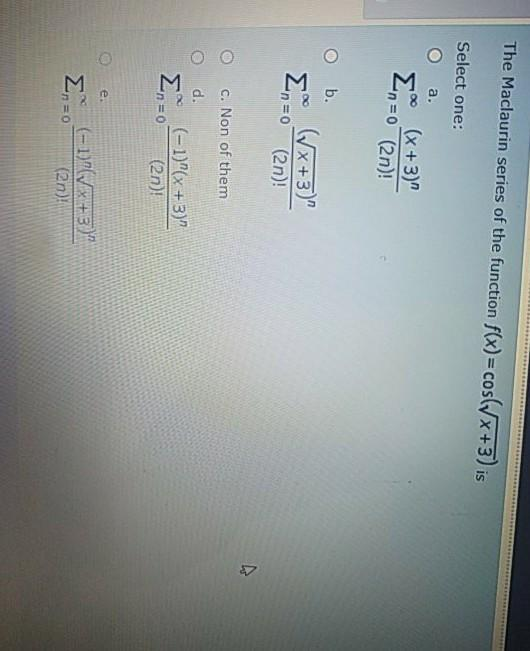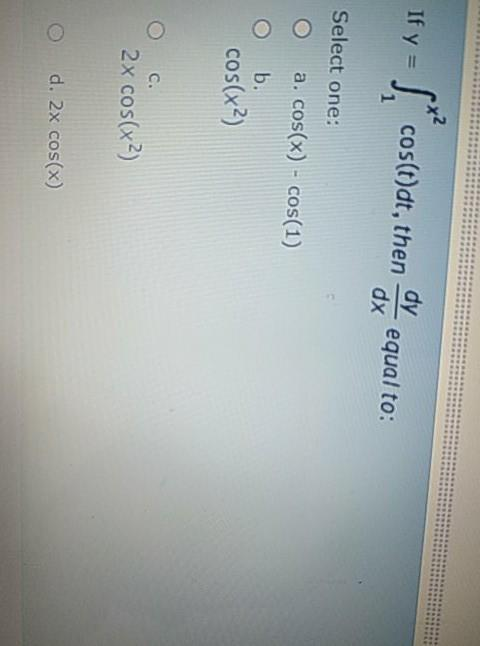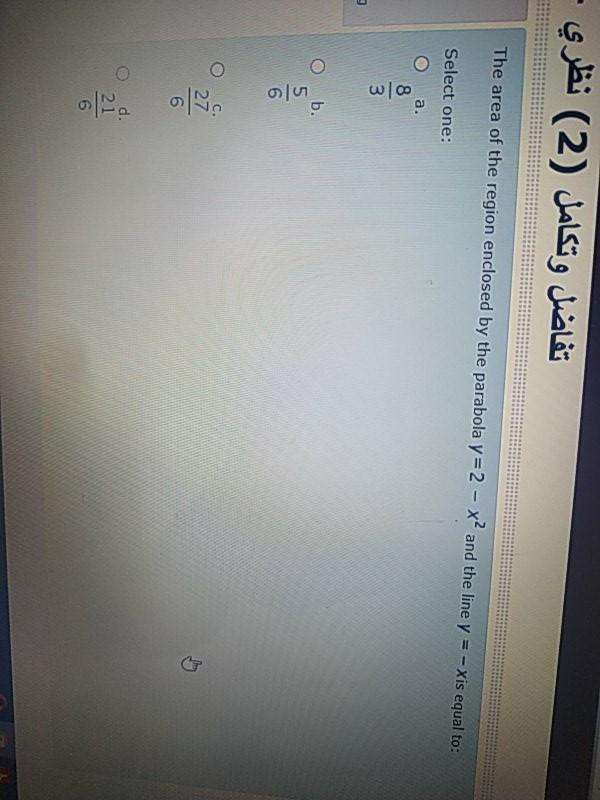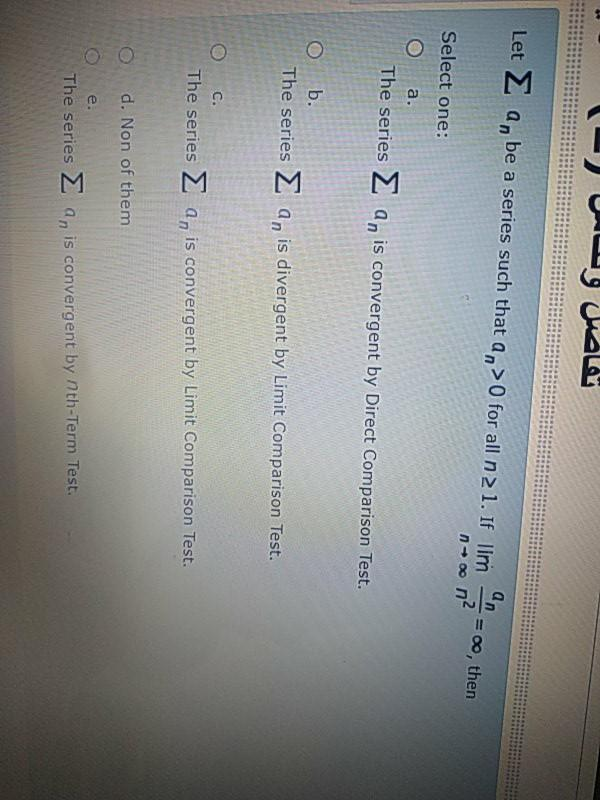### Create an Account

Home / Questions / The Maclaurin series of the function f(x) = cos(x+3) is Select one: a. (x + 3)" n=0 (2n)! ...

# The Maclaurin series of the function f(x) = cos(x+3) is Select one: a. (x + 3)" n=0 (2n)! O b. (Vx+3)" (2n)! n=0 c. Non of them d. (-1)"(x + 3)" (2n)! n=0 e. (-1) (Vx+3) (en) If y = y= Sacostedt , the

The Maclaurin series of the function f(x) = cos(x+3) is Select one: a. (x + 3)" n=0 (2n)! O b. (Vx+3)" (2n)! n=0 c. Non of them d. (-1)"(x + 3)" (2n)! n=0 e. (-1) (Vx+3) (en)
If y = y= Sacostedt , then cos(t)dt, then dy equal to: dx Select one: a. cos(x) - cos(1) O b. cos(x2) C. 2x cos(x2) d. 2x cos(x)
تفاضل وتكامل (2) نظري The area of the region enclosed by the parabola y=2 - x and the line y = - Xis equal to: Select one: : a, 8 3 د b. 5 O 27 6 d. 21 5
تفاضل والات Let Σ an be a series such that an >0 for all n 2 1. If lim An - 0, then noon² Select one: a. The series a, is convergent by Direct Comparison Test. O b. The series 2 a, is divergent by Limit Comparison Test. O C. The series 2 a, is convergent by Limit Comparison Test. d. Non of them e. The series an is convergent by nth-Term Test.Apr 25 2021 View more View LessSubscribe To Get Solution